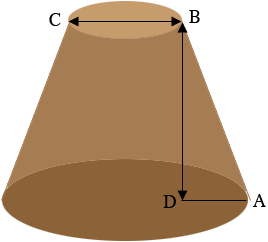SEARCH HOMEMath Central Quandaries & QueriesQuestion from Summer: A sandcastle is in the shape of a truncated cone as shown. Calculate the length of the diameter of the base. The diameter of the top of the truncated cone is 20cm The height is 30cm The slopes are 32cmHi Summer,

I can help get you started.

You didn't include a diagram but I expect it looks something likeThe point $D$ is on the base of the sand castle directly below B so that the angle $NDA$ is a right angle.

The length of $BC$ is 20 cm, the length of $BD$ is 30 cm and the length of $AN$ is 34 cm. Since triangle $ABD$ is a right triangle you can use Pythagoras Theorem to find the length of $DA.$

PennyMath Central is supported by the University of Regina and The Pacific Institute for the Mathematical Sciences.